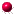XMM-Newton Science Analysis System

eposcorr (eposcorr-3.13) [xmmsas_20211130_0941-20.0.0]

## Statistical method

The statistic for optimizing the match between optical and X-ray sources is:(1)
withthe distance between an X-ray (i) and an optical source (j),the associated error andresp.the number of X-ray sources and optical sources in the list. In the eposcorr task only those optical sources are considered which are withinof an X-ray source (for a given position offset).

In the following we will assume that the errors in the RA and DEC are equal and uncorrelated and follow a Gaussian distribution, we can then write. For the expectation value offor a given X-ray source we get:(2)
The associated variance inis:(3)

Of course, in practice there will be chance coincidences. For chance coincidences the chance that an optical counter part will be within a distancefrom the source is(i.e. within an error circle of). This gives for the expected valuefor a chance coincidence:(4)
And for the variance in:(5)

The number of chance coincidences can be estimated using poison statistics with a poison parameter of, withthe average number of optical sources per unit area. The expected number of sources is thus(where the subscriptdenotes values for each X-ray source, thus allowing for fluctuations in the number of optical sources per area). The expected value foris:(6)

How many counterparts do we need to discriminate between chance coincidences or real counter parts? This question is not easy to answer, as eposcorr optimizesand also for the number of counter parts. This means that Poissonian statistics may not be valid. To get at least an approximate answer, we equate:(7)
or,(8)
The solution of this equation is. I therefore propose to use this number plus the number of degrees of freedom as the minimum threshold for accepting a result of eposcorr. This means that when offsets and in RA and DEC are corrected for the minimum number of optical counter parts should be 7, including a rotational correction this will be 8. This number will be contained in the keyword NMATCHES.

XMM-Newton SOC -- 2021-11-30